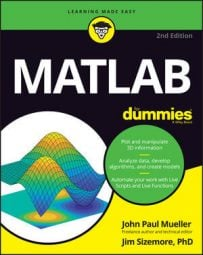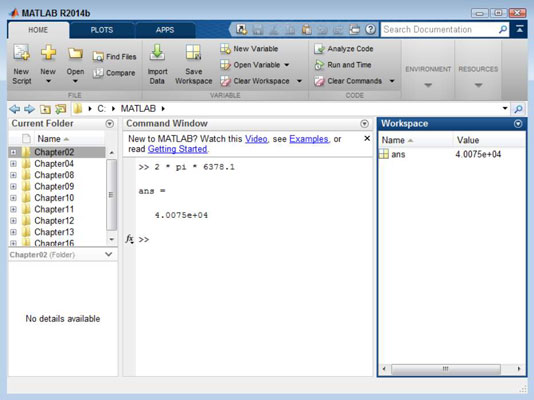##### MATLAB For DummiesMATLAB performs math tasks incredibly well. Sometimes people get so caught up in “what else” an application can do that they miss the most interesting facts that are staring them right in the face. One of the most important things you can do in MATLAB performs is enter formulas.

## Entering a formula

To enter a formula, you simply type it. For example, if you type 2 + 2 and press Enter, you get an answer of 4. Likewise, if you type 2 * pi * 6378.1 and press Enter, you get the circumference of the earth in km (here’s a list of Earth statistics, including radius).

The second formula uses a predefined constant, pi, which equals 3.1416. MATLAB actually defines a number of predefined constants that you can use when entering a formula:

• ans: Contains the most recent temporary answer. MATLAB creates this special temporary variable for you when you don’t provide a variable of your own.

• eps: Specifies the accuracy of the floating-point precision (epsilon), which defaults to 2.2204e-16.

• i: Contains an imaginary number, which defaults to 0.0000 + 1.0000i.

• Inf: Defines a value of infinity, which is any number divided by 0, such as 1 / 0.

• NaN: Specifies that the numerical result isn’t defined (Not a Number).

• pi: Contains the value of pi, which is 3.1416 when you view it onscreen. Internally, MATLAB stores the value to 15 decimal places so that you’re assured of accuracy.

Whenever you type a formula and press Enter, you get an output that specifies the value of ans, which is a temporary value that holds the answer to your question. For example, try typing 2 * pi * 6378.1 and pressing Enter. You see the circumference of the Earth.

## Copying and pasting formulas

With MATLAB, you can copy and paste formulas that you create into other documents (such as a script or function file, or to another application). To begin, you highlight the information you want to copy. Use one of these methods to copy the text after you highlight it:• Click Copy on the QAT.

• Right-click the highlighted text and choose Copy from the context menu.

• Rely on a platform-specific method of copying the text, such as pressing Ctrl+C on Windows.

When you have the text on the Clipboard, you can paste it wherever you want. If you want to paste it somewhere in MATLAB, click wherever you want to put the text, such as after the prompt. Use one of these methods to paste the text:

• Click Paste on the QAT.

• Right click the insertion point and choose Paste from the context menu.

• Rely on a platform-specific method of pasting text, such as pressing Ctrl+V on Windows.